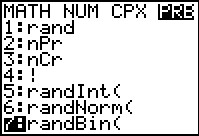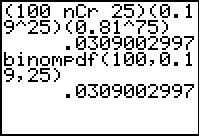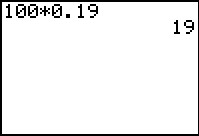# Activities

••• ##### Subject Area

• Math: Statistics: Normal Distributions
• Math: Statistics: Probability and Random Variables

• ##### Author9-12

20 Minutes

• ##### Device
• TI-83 Plus Family
• TI-84 Plus
• TI-84 Plus Silver Edition
•TI-84 Plus C Silver Edition
•TI-84 Plus CE
• ##### Report an Issue

Makin' It Through The Winter#### Activity Overview

Students simulate a binomial distribution and calculate probabilities for a variety of situations involving binomial probability distributions.

#### Key Steps

•Students investigate a binomial distribution of the survival rate of Emperor penguin eggs by creating a list of 50 simulations using the Random Binomial, randBin, command. This effectively simulates data with a given probability of success. Then students will sort the data to determine the experimental probability for a given number of eggs surviving.

•Students calculate theoretical probabilities of a single event using both a formula and the Binomial Distribution Probability, binompdf, command.

•Students calculate theoretical probabilities for at least and at most of a specific x-value using the Cumulative Binomial Probability, binomcdf, command. For example, the probability that at least 25 eggs hatch. They will also calculate the expected value.

Homework problems and their solutions are included in this activity.